# OECD NEET Data Analysis 6- Heteroskedasticity-Robust Inference using RPhoto by Gabriel Garcia Marengo on Unsplash

This post is following of the above post.

Hello. In this post, I will do Heteroskedasiticity test usung R.

In te previous post, I meade regression model using R.

Let's plot fitted value vs. residual value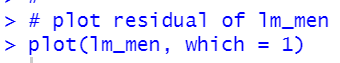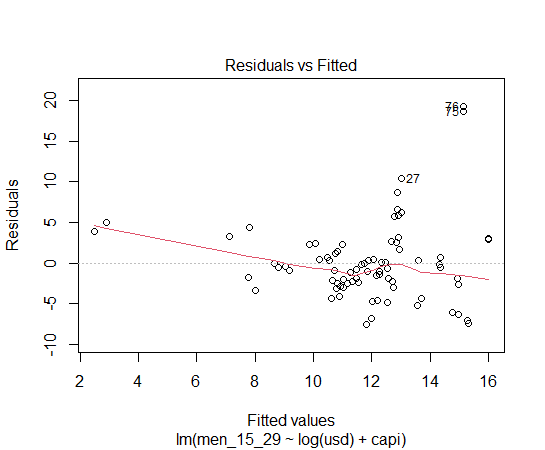Above plot is men_15_29 ~ log(usd) + capi.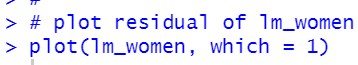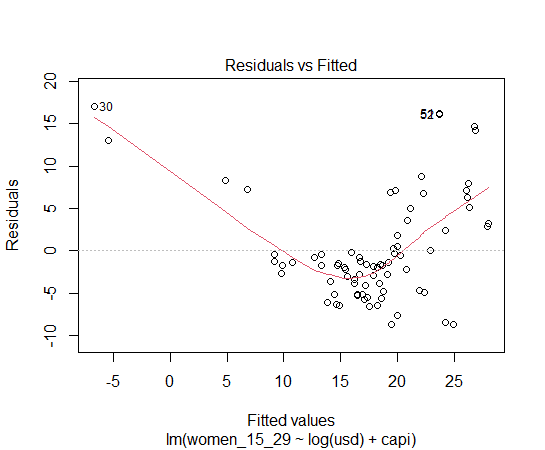Above plot is for women_15_29 ~ log(usd) + capi.

Let's test if those models have heteroskedasticity.
FIrstly, I use Breush-Pegan test manually.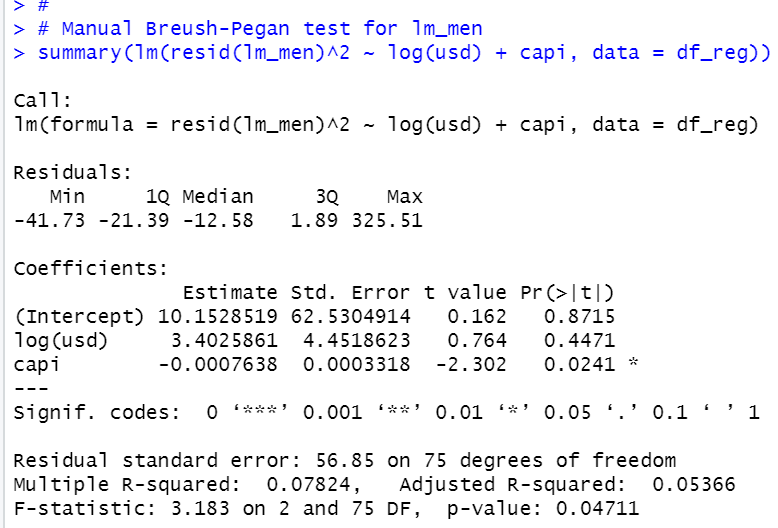p-value is 0.04711, which is smaller than 0.05. So, lm_men model has heteroskedasticity.

Next, let's use lmtest package and bptest() function.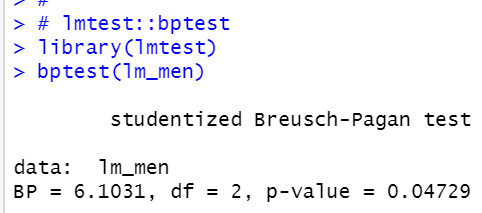p-value is 0.04729, it is close to manual calculation.

Then, let's check for lm_women. I use manual Special Case of White Test.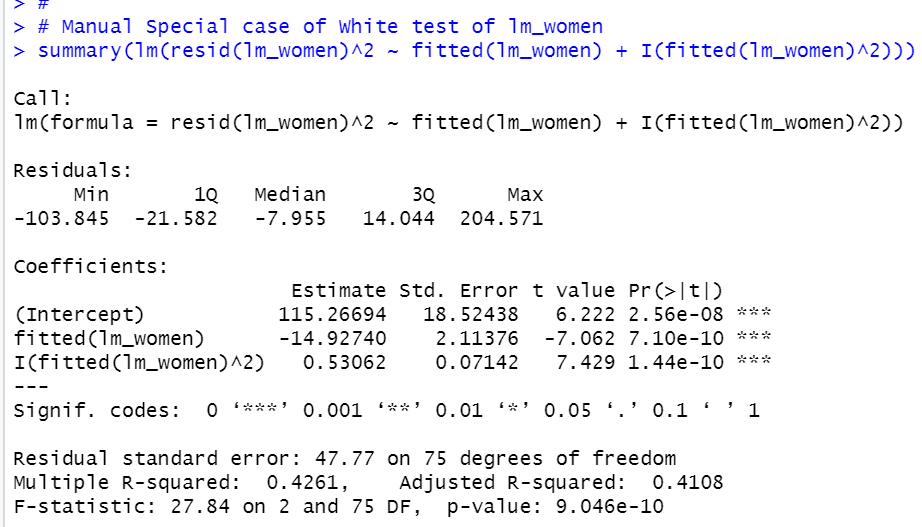P-value is almost 0. So, lm_women model has heteroskedasticiy.

SInce the both model, lm_men and lm_women have heteroskedasticity, let's see heteroskedasticiry-robust standard error.

I use car package and lmtest package, then use coeftest() function.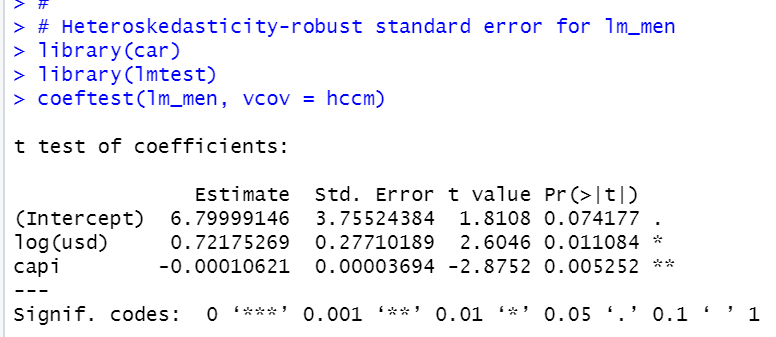On Heteroskedasticity-robust standard error, log(usd) and capi are statistically significant.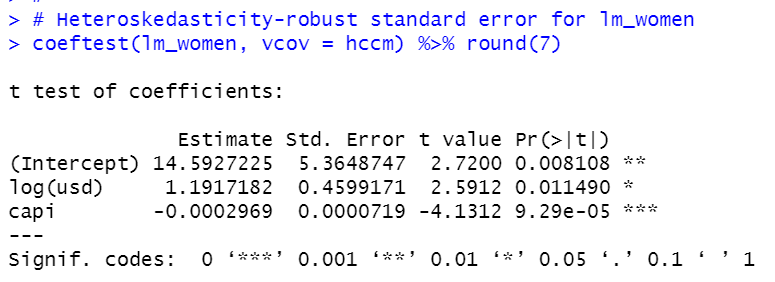log(usd) and capi are statistically significant on lm_women model under heteroskedasticity robust inference.

Thant's it. Thank you!

Next post is,

To read from the 1st post,